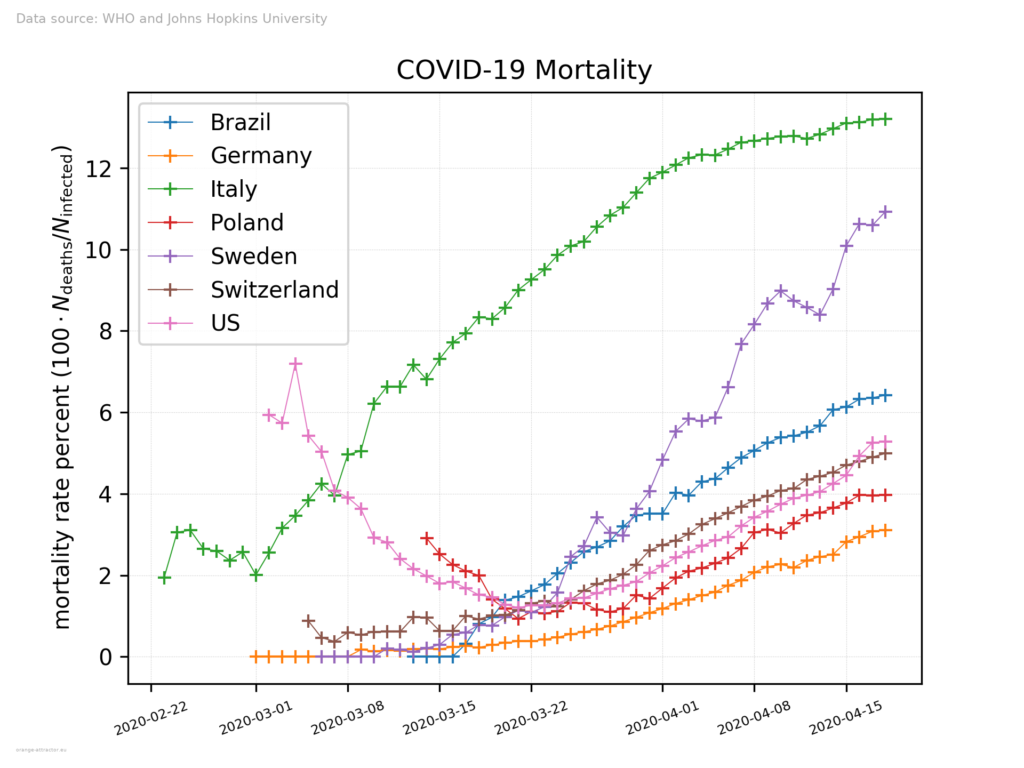# COVID-19 Death Rate

We present death rate versus time chart for selected countries: Brazil, Germany, Italy, US, Poland, Sweden, Switzerland. Death rate is calculated as follows:
$$d = \frac{N_\mathrm{deaths}}{N_{\mathrm{infected}}} \cdot 100,$$
where $$N_\mathrm{deaths}$$ is number of deaths caused by COVID-19 infection since the beginning of epidemic, $$N_{\mathrm{infected}}$$ is a total number of infected people.
For each country, the plot starts at the first date where there are at least 100 infected cases, which is common practice.

The base data for the chart are the official data published by WHO and provided by John Hopkins University: https://github.com/CSSEGISandData/COVID-19